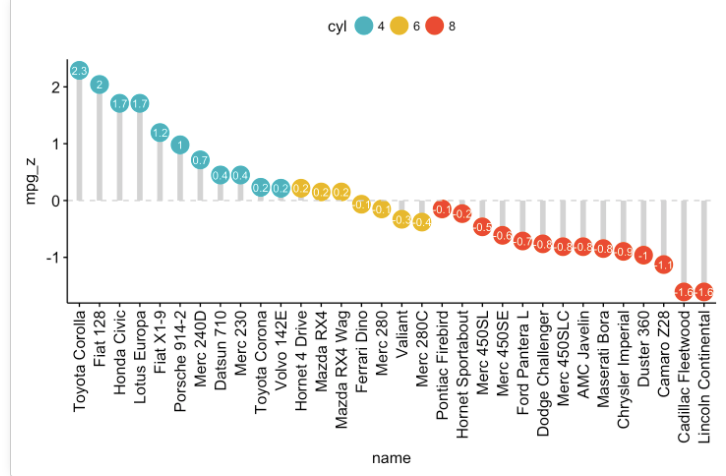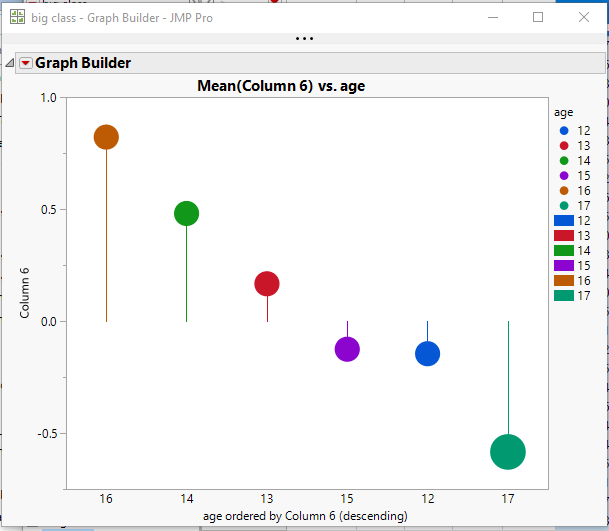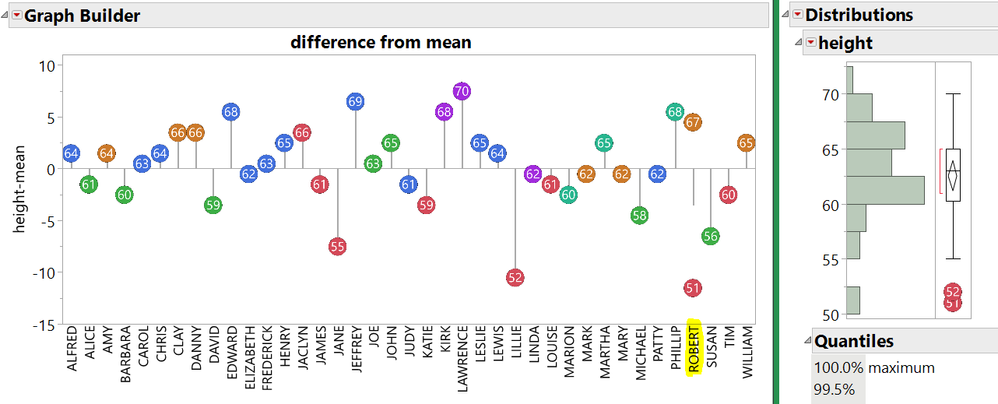Our World Statistics Day conversations have been a great reminder of how much statistics can inform our lives. Do you have an example of how statistics has made a difference in your life? Share your story with the Community!
Choose Language Hide Translation Bar
Highlighted

## Lollipop graph

Is it possible via GraphBuilder to make  a lollipop plot? See example I've posted. I know you can get something akin to this when looking at time series data (under the time series platform). The control chart very nearly does this, too, but it is obviously to be used for other sorts of analyses, and the lines are not drawn directly below the points. What I want is for the point plotted to have a line drawn above or below it that connects it to the mean (to emphasize deviation from the mean). I feel like all the elements for making such a plot are there!Anderson B. Mayfield
1 ACCEPTED SOLUTION

Accepted Solutions
Highlighted

## Re: Lollipop graph

Here is a graph using the Big Class data table, with an added column that has positive and negative random values.And here is the script

``````Graph Builder(
Size( 534, 450 ),
Show Control Panel( 0 ),
Variables(
X( :age, Order By( :Column 6, Descending, Order Statistic( "Mean" ) ) ),
Y( :Column 6 ),
Color( :age )
),
Elements(
Points( X, Y, Legend( 5 ), Summary Statistic( "Mean" ) ),
Bar( X, Y, Legend( 6 ), Bar Style( "Needle" ) )
),
SendToReport(
Dispatch(
{},
"400",
ScaleBox,
{Legend Model(
5,
Properties( 0, {Marker Size( 20 )}, Item ID( "12", 1 ) ),
Properties( 1, {Marker Size( 20 )}, Item ID( "13", 1 ) ),
Properties( 2, {Marker Size( 20 )}, Item ID( "14", 1 ) ),
Properties( 3, {Marker Size( 20 )}, Item ID( "15", 1 ) ),
Properties( 4, {Marker Size( 20 )}, Item ID( "16", 1 ) ),
Properties( 5, {Marker Size( 29 )}, Item ID( "17", 1 ) ),
Properties( -1, {Marker Size( 20 )}, Item ID( "Mean", 1 ) )
)}
)
)
);``````
Jim
9 REPLIES 9
Highlighted

## Re: Lollipop graph

Here is a graph using the Big Class data table, with an added column that has positive and negative random values.And here is the script

``````Graph Builder(
Size( 534, 450 ),
Show Control Panel( 0 ),
Variables(
X( :age, Order By( :Column 6, Descending, Order Statistic( "Mean" ) ) ),
Y( :Column 6 ),
Color( :age )
),
Elements(
Points( X, Y, Legend( 5 ), Summary Statistic( "Mean" ) ),
Bar( X, Y, Legend( 6 ), Bar Style( "Needle" ) )
),
SendToReport(
Dispatch(
{},
"400",
ScaleBox,
{Legend Model(
5,
Properties( 0, {Marker Size( 20 )}, Item ID( "12", 1 ) ),
Properties( 1, {Marker Size( 20 )}, Item ID( "13", 1 ) ),
Properties( 2, {Marker Size( 20 )}, Item ID( "14", 1 ) ),
Properties( 3, {Marker Size( 20 )}, Item ID( "15", 1 ) ),
Properties( 4, {Marker Size( 20 )}, Item ID( "16", 1 ) ),
Properties( 5, {Marker Size( 29 )}, Item ID( "17", 1 ) ),
Properties( -1, {Marker Size( 20 )}, Item ID( "Mean", 1 ) )
)}
)
)
);``````
Jim
Highlighted

## Re: Lollipop graph

Jim,

Wow, thanks so much. That's exactly what I wanted. I didn't even think to integrate a "needle" bar graph with a "mean" point sitting on top, but for those who want to do it all through GraphBuilder (i.e., without scripting), that's how you do it. If you additionally want to interactively size the dots in proportion to the response variable of interest, simply drag the parameter to the "size" drop zone on GraphBuilder. I am attaching my first lollipop plot as an additional example. Thanks so much and hopefully this may help others who stumbled across this post.

Anderson B. Mayfield
Highlighted

## Re: Lollipop graph

Another approach, requiring more JSL for custom Markers.Using an image for the marker. Use As Marker will show up in other graphs.

There are two Roberts in Big Class; the Needle is the average. The points are shown for both.

``````dt = Open( "\$sample_data/big class.jmp" );
dt << New Column( "height-mean", formula( height - Col Mean( height ) ) );
dt << Color by Column( :Age );

// build the floating round marker...
dt << New Column( "marker",
expression,
formula(

box = (Graph Box(
// make a small drawing space 100x100 pixels, scaled from -1 to 1. Black background becomes transparent below
framesize( 100, 100 ), X Scale( -1, 1 ), Y Scale( -1, 1 ), <<backgroundcolor( RGB Color( 0, 0, 0 ) ),
// use the row's color. it is pretty dark when filled
Fill Color( Color Of( Row State() ) );
Circle( {0, 0}, .75, "FILL" );
Text Color( RGB Color( 1, 1, 1 ) );//white
Text Size( 40 );// adjust as needed
Text( CenterJustified, {0, -.35/*adjust as needed for v-center*/}, Char( height ) );

));
// trim the frame edges and make a transparent background
{r, g, b} = ((box[FrameBox( 1 )]) << getimage) << getpixels( "rgb" );
r = r[4 :: (N Rows( r ) - 5), 4 :: (N Cols( r ) - 5)];//trim
g = g[4 :: (N Rows( g ) - 5), 4 :: (N Cols( g ) - 5)];//trim
b = b[4 :: (N Rows( b ) - 5), 4 :: (N Cols( b ) - 5)];//trim
a = r + g + b != 0;
Show( r, g, b, a );
New Image( "rgba", {r, g, b, a} );

),
<<useformarker // use this column for markers
);

dt << Graph Builder(
Size( 1200, 500 ),
Show Control Panel( 0 ),
Show Legend( 0 ),
Variables( X( :name ), Y( :Name( "height-mean" ) ) ),
Elements( Bar( X, Y, Legend( 5 ), Bar Style( "Needle" ) ), Points( X, Y, Legend( 7 ) ) ),
SendToReport(
Dispatch(
{},
"height-mean",
ScaleBox,
{Min( -15 ), Max( 11 ), Inc( 5 ), Minor Ticks( 1 ), Add Ref Line( 0, "Solid", "Gray", "", 1 )}
),
Dispatch( {}, "400", ScaleBox, {Legend Model( 5, Properties( 0, {Line Color( 82 )}, Item ID( "Mean", 1 ) ) )} ),
Dispatch( {}, "graph title", TextEditBox, {Set Text( "difference from mean" )} ),
Dispatch( {}, "X title", TextEditBox, {Set Text( "" )} )
)
);``````

Craige
Highlighted

Love it!

Jim
Highlighted

## Re: Lollipop graph

You can actually reproduce this interactively (albeit less beautifully) by increasing the marker size, labeling by height, then reducing the marker transparency (I halved it). In this example, I just colored by gender.

The only issue I can see is that, if you want to have the icon sizes scale with something (like age in this second example) AND have the labels (for height in this example), you may need to manually drag the labels around so that they don't fall in a weird place (see second plot, in which case I manually changed the location of Clay and Danny's labels). There might be a work-around though (I tried messing around with the marker sizing options and failed).

Anderson B. Mayfield
Highlighted

## Re: Lollipop graph

Nice! You can probably order the marker segments so the numbers draw on top of the circles. And you might be able to make the numbers be markers as well (UseForMarker attribute on a column). That would center the number in the circle. Avoiding the JSL in my example would be a good choice; it is pretty slow to make a graph and take its picture for each data point.

Craige
Highlighted

## Re: Lollipop graph

When I run this under v13.2.1 , the lollipops markers are very small. So small the embedded number isn't visible. How can I increase the size of the markers?
Highlighted

## Re: Lollipop graph

The "<<useformarker" message is not known in JMP 13.2.1.  However, you can right click on the graph and select "Custom" and then go to the Marker entry and right click on the marker, and change the marker size.

Jim
Article Labels